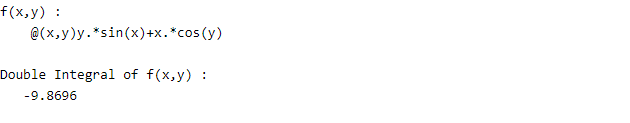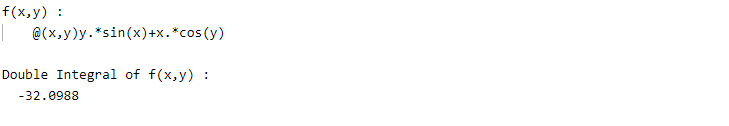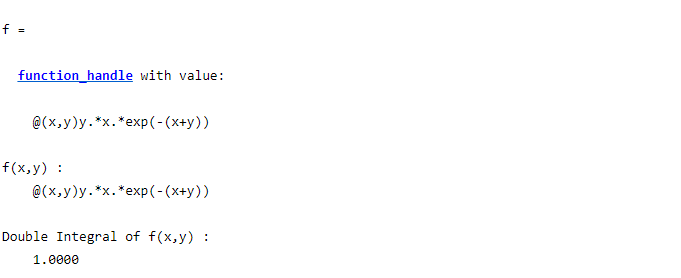# Double Integral in MATLAB

• Last Updated : 08 Mar, 2022

The double integral of a non-negative function f(x, y) defined on a region in the plane tells us about the volume of the region under the graph. The double integral of a function of two variables, f(x, y) over the region R can be expressed as follows :MATLAB allows users to calculate the double integral of a function using the integral2() method. Different syntax of integral2() method are:

• F = integral2(fun,xmin,xmax,ymin,ymax)
• F = integral2(fun,xmin,xmax,ymin,ymax,Name,Value)

#### Syntax:

 integral2(fun,xmin,xmax,ymin,ymax)

This function approximates the integral of any function f = fun(x,y) in the region xmin ≤ x ≤ xmax and ymin(x) ≤ y ≤ ymax(x)

Example 1:

## Matlab

 % MATLAB Code to % Create a function in x and y% Function : y*sin(x) + x*cos(y)f = @(x,y) y.*sin(x)+x.*cos(y);disp("f(x,y) :");disp(f);  % Double Integral of f(x)% over pi≤x≤2*pi and 0≤y≤pid = integral2(f,pi,2*pi,0,pi);disp("Double Integral of f(x) :");disp(d);

Output :Example 2:

## Matlab

 % MATLAB Code to% Create a function in x and y% Function : y*sin(x) + x*cos(y)f = @(x,y) y.*sin(x)+x.*cos(y);disp("f(x,y) :");disp(f);  % Double Integral of f(x)% over pi≤x≤2*pi and 0≤y≤xymax = @(x) x;d = integral2(f,pi,2*pi,0,ymax);disp("Double Integral of f(x) :");disp(d);

Output :#### Syntax:

 F = integral2(fun,xmin,xmax,ymin,ymax,Name,Value)

It specifies additional options with one or more Name, Value pair arguments. The most popular Name used is ‘Method’

The Possible values are:

• tiled: The limits for the integration must be finite for this method to work. It subdivides the integration zone into simpler rectangular areas if needed after transforming it to a rectangular shape.
• iterated: In this method, iterated integration is applied. It is the process of repeatedly integrating the results of the previous integrations when the function consists of more than one variable. In the function. In this method, the integration limits can be infinite.
• auto: The ‘auto’ method is used as the default value. It chooses tiled or iterated based on the limits chosen. If limits are infinite then it chooses ‘iterated’ otherwise it calculates using the ’tiled’ method.

Example 3:

## Matlab

 % MATLAB Code to% Create a function in x and y% Function : y*sin(x) + x*cos(y)f = @(x,y) y.*sin(x)+x.*cos(y);disp("f(x,y) :");disp(f);  % Double Integral of f(x) using Tiled Method% over pi≤x≤2*pi and 0≤y≤pid = integral2(f,pi,2*pi,0,pi,'Method','Tiled');disp("Double Integral of f(x) :");disp(d);

Output :Example 4:

## Matlab

 % MATLAB code for iterated % Create a function in x and y% Function : x*y*e^-(x+y)f = @(x,y) y.*x.*exp(-(x+y))disp("f(x,y) :");disp(f);  % Double Integral of f(x) using Iterated method% over 0≤x≤Inf and 0≤y≤Infd = integral2(f,0,Inf,0,Inf,'Method','Iterated');disp("Double Integral of f(x) :");disp(d);

Output:My Personal Notes arrow_drop_up